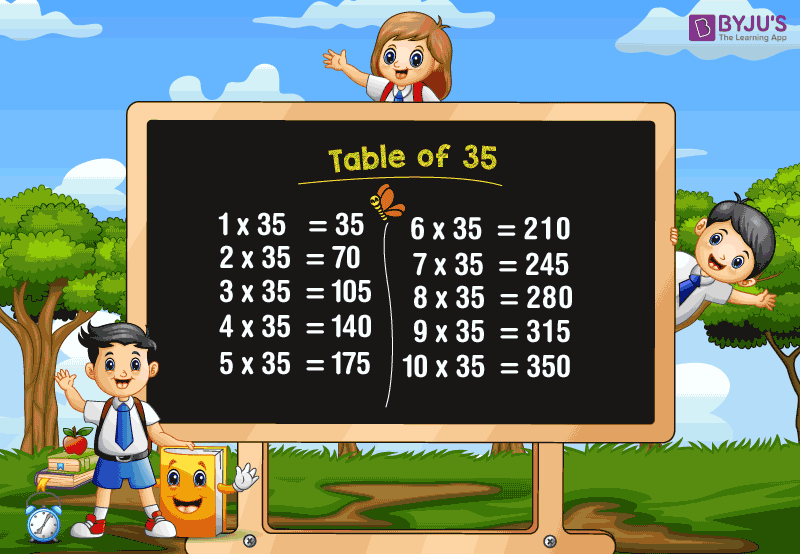# Table of 35

Table of 35 is Maths table of multiplication. This table is used to perform mathematical computations. Students can use this table to solve problems based on multiplication. Check Tables from 1 to 100 for more details.35 times of a number represents that 35 is repeatedly added as per that number. For example, 35 times of 4 represents 35 been added to itself 4 times.

35 + 35 + 35 + 35 = 140

Thus, in the form of multiplication, we can write,

35 x 4 = 140

In the same way, let us see the multiplication of thirty-five with other numbers (from 1 to 20) with the help of the chart given below.

## Multiplication Table of 35

 35 x 1 = 35 35 x 2 = 70 35 x 3 = 105 35 x 4 = 140 35 x 5 = 175 35 x 6 = 210 35 x 7 = 245 35 x 8 = 280 35 x 9 = 315 35 x 10 = 350 35 x 11 = 385 35 x 12 = 420 35 x 13 = 455 35 x 14 = 490 35 x 15 = 525 35 x 16 = 560 35 x 17 = 595 35 x 18 = 630 35 x 19 = 665 35 x 20 = 700

## 35 Times Table

Read the thirty-five times table here.

• One time thirty-five is 35
• Two times thirty-five is 70
• Three times thirty-five is 105
• Four times thirty-five is 140
• Five times thirty-five is 175
• Six times thirty-five is 210
• Seven times thirty-five is 245
• Eight times thirty-five is 280
• Nine times thirty-five is 315
• Ten times thirty-five is 350

## Frequently Asked Questions on Table of 35

### What is the table of 35?

Table of 35 gives the repeated addition of number 35, when multiplied by another number. For example, 35 x 2 = 70, i.e. 35+35 = 70.

### What is the value of 5 times 35?

5 times of 35 is equal to 175

### What is the value of 30 times 35?

Thirty times thirty-five is equal to 1050.

### Write the first five multiples of 35.

First five multiples of 35 are:

35, 70, 105, 140 and 175

### What is the value of 2 (35 x 8)?

2 (35 x 8)

Since, 35 x 8 = 280

Therefore,

2 x 280

= 560